Area + fractions - examples

1. Cone A2VSurface of cone in the plane is a circular arc with central angle of 126° and area 415 cm2. Calculate the volume of a cone.
2. ConeCircular cone of height 15 cm and volume 5699 cm3 is at one-third of the height (measured from the bottom) cut by a plane parallel to the base. Calculate the radius and circumference of the circular cut.
3. FloorThe floor area of ​​the room is 31 m2 and has a width of 4.3 m. How many centimeters of circumference measured floor on the map at the scale 1:75?
4. Above EarthTo what height must a boy be raised above the earth in order to see one-fifth of its surface.
5. Forest nursery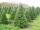In Forest nursery plant one pine to 1.9 m2. Calculate how many plants are planting in area 362 acres.
6. Three shapes1/5 of a circle is shaded. The ratio of area if square to the sum of area of rectangle and that of the circle is 1:2. 60% of the square is shaded and 1/3 of the rectangle is shaded. What is the ratio of the area of circle to that of the rectangle?
7. Mr. Happy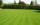Mr. Happy planted 36.6 meters square gardens grass; It's a third of the garden more than half of the garden. What is square area of this garden?
8. Garden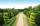The garden has a rectangular shape with lengths of 25 and 40 meters. It has been expanded so that each of its size increased by one fifth. How many square meters increased its acreage?
9. Oceans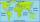The Earth's surface is approximately 510,000,000 km2 and is 7/10 covered by oceans. Of which 1/2 covers the Pacific Ocean, the Atlantic Ocean 1/4, the Indian Ocean 1/5 and the Arctic Ocean 1/20. What parts of the Earth's surface cover each ocean?
10. Right triangle from axesA line segment has its ends on the coordinate axes and forms with them a triangle of area equal to 36 sq. Units . The segment passes through the point ( 5,2). What is the slope of the line segment. ?
11. Two brothersThe two brothers were to be divided according to the will of land at an area of 1ha 86a 30m2 in a ratio of 5:4. How many will everyone get?
12. Hamster cage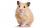Ryan keeps his hamster cage on his dresser. The area of the top of Ryan's dresser is 1 2\3 as large as the area of the bottom of his hamster cage. The area of the dresser top is 960 square inches. How many square inches of his dresser top are not covered b
13. GardenThe rectangular garden has dimensions of 27 m and 30 m. Peter and Katka split it in a ratio of 4:5. How many square meters did Katkin measure part of the garden?
14. Irrigation sprinklerThe irrigation sprinkler can twist with an angle of 320° and has a reach of 12 meters. Which area can irrigate?
15. In theIn the national park, the ratio of the wooded area to grassland is 4: 1. The total area is 385km2. What area is wooded?
16. Sewing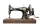The lady cut off one half of cloth. She needed three-quarters of this piece to sew a skirt. What part of the original piece of cloth still remained?
17. Three segments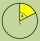The circle is divided into 3 segments. Segment A occupies 1/4 of the area, segment B occupies 1/3 of the area. What part is occupied by section C? In what proportion are areas A: B: C?
18. Fe metal sheetFor one product, 5/8 of the metal sheet are consumed, to the second 5/6 of remains. What part of the sheet metal is consumed for both products together?
19. Reducing scale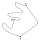I am sizing some landscaping and I measured everything for a 20 to 1 scale. I realized when I was done that it was at 30 to 1. How can I convert what I already have to 30 to 1. (Example, grass area is 22,871.6' at 20 to 1, how do you figure it out to be 30
20. Land area 2A land area was divided among the three heirs in the ratio 5:2:4. If the largest share was 20 hectares of land, what is the total area of land? Please show your solution and what kind of proportion is this please

Do you have an interesting mathematical example that you can't solve it? Enter it, and we can try to solve it.

To this e-mail address, we will reply solution; solved examples are also published here. Please enter e-mail correctly and check whether you don't have a full mailbox.

Need help calculate sum, simplify or multiply fractions? Try our fraction calculator.